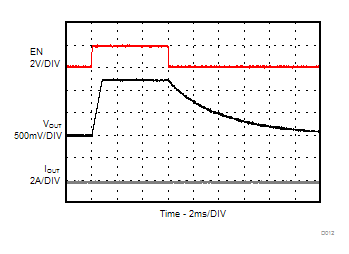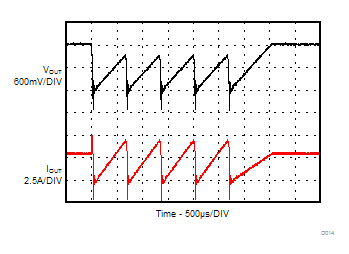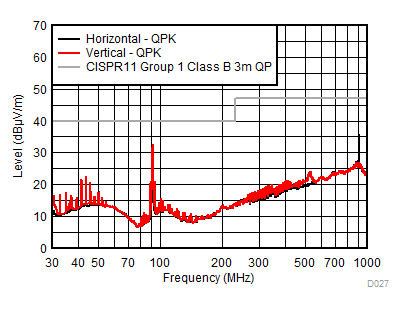SLVSCN4D October   2014  – June 2019

PRODUCTION DATA.

1. Features
2. Applications
3. Description
1.     Device Images
4. Revision History
5. Pin Configuration and Functions
6. Specifications
7. Detailed Description
1. 7.1 Overview
2. 7.2 Functional Block Diagram
3. 7.3 Feature Description
4. 7.4 Device Functional Modes
8. Application and Implementation
1. 8.1 Application Information
2. 8.2 Typical Applications
1. 8.2.1 1.2-V Output Application
9. Power Supply Recommendations
10. 10Layout
11. 11Device and Documentation Support
12. 12Mechanical, Packaging, and Orderable Information

#### Package Options

Refer to the PDF data sheet for device specific package drawings

• SIL|8

#### 8.2.1.3 Application Performance Curves

TA = 25°C, VIN = 5 V, VOUT = 1.2 V, BOM = Table 3 unless otherwise noted.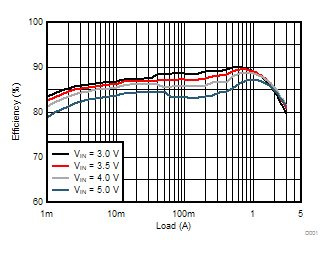VOUT = 1.2 V
Figure 6. Efficiency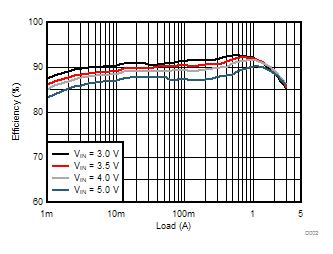VOUT = 1.8 V
Figure 8. Efficiency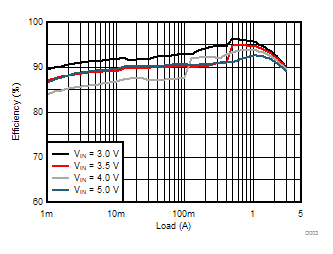VOUT = 2.6 V
Figure 10. Efficiency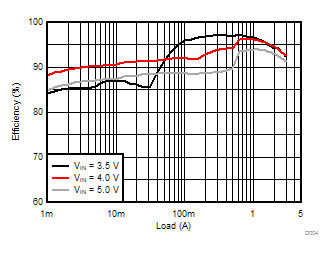VOUT = 3.3 V
Figure 12. Efficiency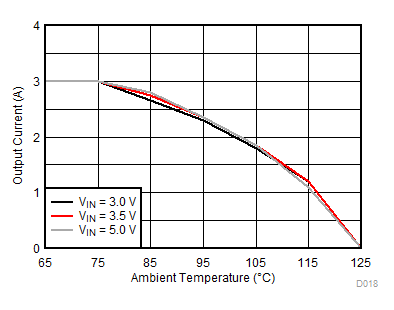VOUT = 1.2 V θJA = 46.6°C/W
Figure 14. Thermal Derating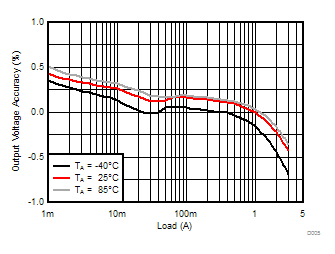IOUT = 2 A
Figure 18. Input and Output Ripple in PWM Mode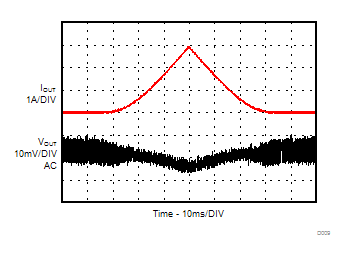IOUT = 25 mA to 3 A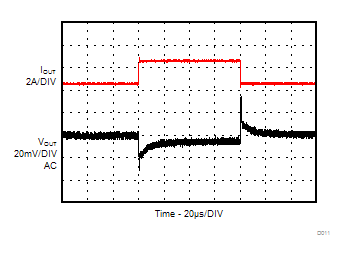IOUT = 0.5 A to 2.5 A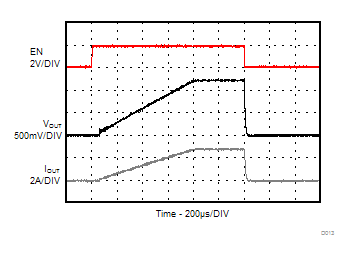Figure 24. Startup / Shutdown with Resistive Load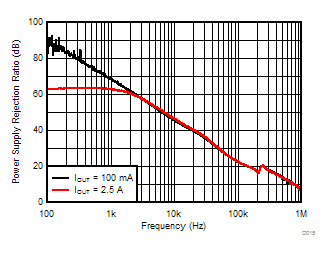Figure 26. Power Supply Rejection Ratio (PSRR)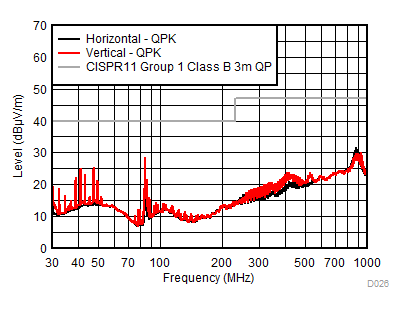RLOAD = 0.47 Ω, VIN = 5 V (battery supply), VOUT = 1.2 V, EMI test board without filters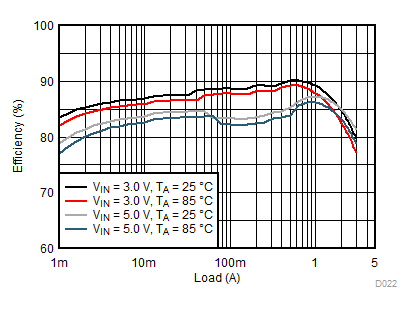VOUT = 1.2 V
Figure 7. Efficiency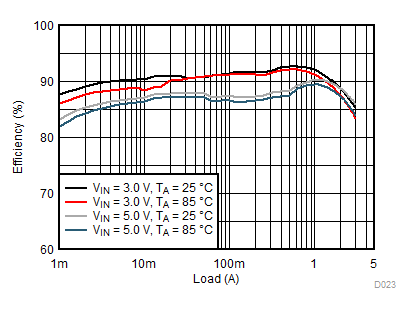VOUT = 1.8 V
Figure 9. Efficiency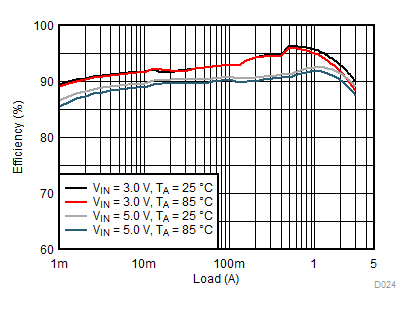VOUT = 2.6 V
Figure 11. Efficiency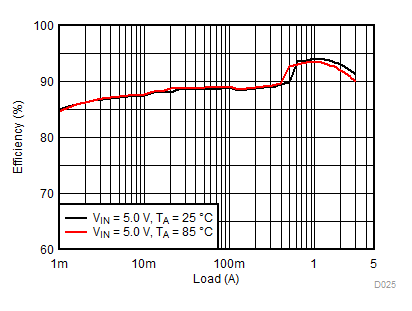VOUT = 3.3 V
Figure 13. Efficiency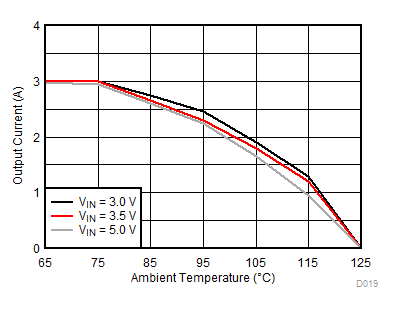VOUT = 2.6 V θJA = 46.6°C/W
Figure 15. Thermal Derating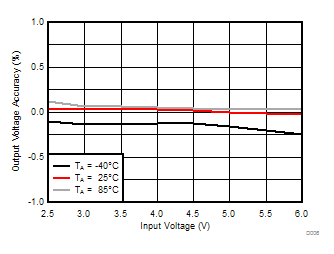IOUT = 1 A
Figure 17. Line Regulation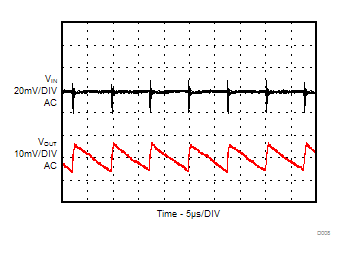IOUT = 25 mA
Figure 19. Input and Output Ripple in PSM Mode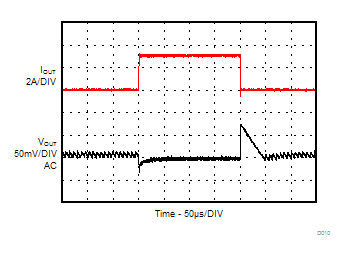IOUT = 25 mA to 3 A